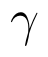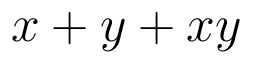Select Page

# CBSE Maths 12 Science MCQ Three Dimensional Geometry Solutions in English

CBSE Maths 12 Science MCQ Three Dimensional Geometry Solutions in English to enable students to get Solutions in a narrative video format for the specific question.

Expert Teacher provides CBSE Maths 12 Science MCQ Three Dimensional Geometry Solutions through Video Solutions in English language. This video solution will be useful for students to understand how to write an answer in exam in order to score more marks. This teacher uses a narrative style for a question from Three Dimensional Geometry not only to explain the proper method of answering question, but deriving right answer too.

Please find the question below and view the Solution in a narrative video format.

Question:

Solution Video in English:

## Similar Questions from CBSE, 12th Science, Maths, Three Dimensional Geometry

Question 1 : What is the distance of the point (p, q, r) from the x-axis ? (View Answer Video)

Question 2 : Find the distance between the point (7, 2, 4) and the plane determined by the points A(2, 5, -3), B(-2, -3, 5) and C(5, 3, -3). (View Answer Video)

Question 3 : Find the distance of the point (2, 12, 5) from the point of intersection of the linesand the plane(View Answer Video)

Question 4 : A line passes through the point with position vectorand makes anglesandwith x, y and z-axis respectively. Find the equation of the line in the Cartesian form. (View Answer Video)

Question 5 : If a line makes angleandwith the coordinate axis, then find the value of(View Answer Video)

### Inverse Trigonometric Functions

Question 1 : Solve for(View Answer Video)

Question 2 : If, then write the value of. (View Answer Video)

Question 3 : Solve the equation :(View Answer Video)

Question 4 :is equal to :

Question 5 : Writein the simplest form. (View Answer Video)

### Linear Programming

Question 1 : The objective function is maximum or minimum, which lies on the boundary of the feasible region. (View Answer Video)

### Differential Equations

Question 1 : Form the differential equation of the family of circles in the second quadrant and touching the co-ordinate axes. (View Answer Video)

Question 2 : Solve the differential(View Answer Video)

Question 3 : Find the solution of the differential equation(View Answer Video)

Question 4 : Write the degree of the differential equation(View Answer Video)

Question 5 : Solve the differential equation(View Answer Video)